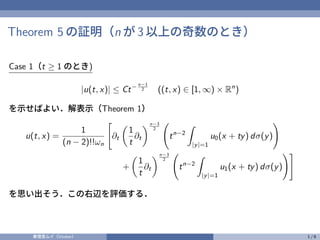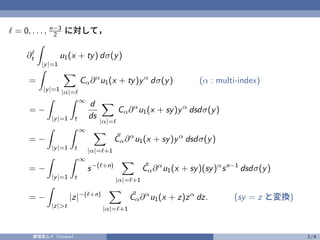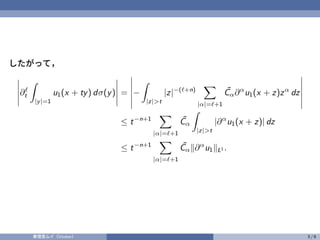Successfully reported this slideshow.×

# ndwave8.pdf

The proof of pointwise estimates of solutions to the wave equation in odd (greater than or equal to 3) space dimensions. (in Japanese)

The proof of pointwise estimates of solutions to the wave equation in odd (greater than or equal to 3) space dimensions. (in Japanese)